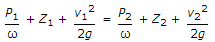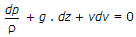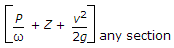# Civil Engineering - Hydraulics

### Exercise :: Hydraulics - Section 1

46.

Pick up the correct statement regarding Borda's mouth piece running full from the following :

 A. Actual velocity at the out let is gH B. Theoretical velocity at the outlet is 2gH C. Coefficient of velocity is 1/2 D. Coefficient of contraction is 1 E. All the above.

Answer: Option E

Explanation:

No answer description available for this question. Let us discuss.

47.

Differential manometers are used to measure

 A. pressure in water channels, pipes, etc. B. difference in pressure at two points C. atmospheric pressure D. very low pressure.

Answer: Option B

Explanation:

No answer description available for this question. Let us discuss.

48.

A tank 4m x 3m x 2m containing an oil of specific gravity 0.83 is moved with an acceleration g/2 m sec2. The ratio of the pressures at its bottom when it is moving vertically up and down, is

 A. 2 B. 3 C. 1/2 D. 1/3

Answer: Option B

Explanation:

No answer description available for this question. Let us discuss.

49.

The most familiar form of Bernoulli's equation, is

 A.B.C.= constant head D. none of these.

Answer: Option C

Explanation:

No answer description available for this question. Let us discuss.

50.

Highest dam in India, is

 A. Bhakra dam B. Hirakund dam C. Nagarjuna Sagar dam D. Iddiki dam.

Answer: Option A

Explanation:

No answer description available for this question. Let us discuss.

#### Current Affairs 2021

Interview Questions and Answers